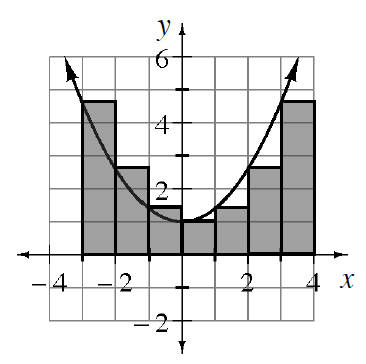### Home > APCALC > Chapter 1 > Lesson 1.2.2 > Problem1-36

1-36.

The graph of $j(x)=\frac{2}{5}x^2+1$ is shown at right. .

1. Approximate the area under the curve for $−3\le x\le3$ by calculating the sum of the areas of the six left endpoint rectangles as shown. (The height of a left endpoint rectangle is determined by the function’s value at the left $x$-value.)

Notice that the right-most rectangle shown in the picture is not included in the given domain $−3\le x\le3$.

The area of each rectangle can be determined by $A=bh$ The base, $b$, of each rectangle is $1$.The height, $h$, of each rectangle can be determined by the function: $y=(0.4)x^2+1$.

$A=(1)(4.6)+(1)(2.6)+(1)(1.4)+(1)(1)+(1)(1.4)+(1)(2.6)=$ __________

2. Is the approximation in part (a) too high or too low? How can you tell?

This is a hard one to visualize. Remember to consider only domain $−3\le x\le3$. Does it appear that the rectangles contain more area than the area under the parabola or less?

3. Now, sketch this function with six right endpoint rectangles and compute the approximate area.

$A = (0.4(−3)^2 + 1) + (0.4(−2)^2 + 1) + (0.4(−1)^2 + 1) + (0.4(0)^2 + 1) + (0.4(1)^2 + 1) + (0.4(2)^2 + 1) = 13.6$

4. You should have obtained the same answers using right and left endpoint rectangles. Will this be true for all functions? If so, explain why. If not, explain what was special about this case that made the area estimates equal. Give an example of a case where the area estimates will be different.

Consider the symmetry of the parabola on the given domain.

Use the eTool below to view the graphs.
Click the link at right for the full version of the eTool:. Calc 1-36 HW eTool